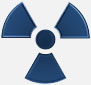Buying A Geiger Counter - Page 7

How to read a Geiger Counter

How to read a Geiger Counter

The Geiger counter measures the radiation levels in different ways. Analog meters can provide a reading in Counts Per Minute (CPM) and their equivalent Radiation Level. For example lets look at the meter face to a common analog Geiger counter, see figure 12.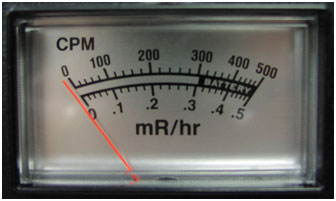At its most sensitive scale a count of 500 CPM is equivalent to a radiation level of 0.5 mR/hr. This model Geiger counter has a 10X and 100X switch to select ranges from 0.5 mR/hr to 5 mR/hr and 50 mR/hr respectively.

To obtain the Counts Per Second (CPS) divide the CPM by 60. So a CPM reading of 300 is equal to 5 CPS (300/60 = 5).

Digital Geiger Counters

Digital Geiger Counters have a digital display. The digital display has a number of advantages, such as providing an accurate and exact count of the detected radioactive particles in either the CPS or CPM measurement and their equivalent radiation level. The analog display approximates the number of clicks per minute.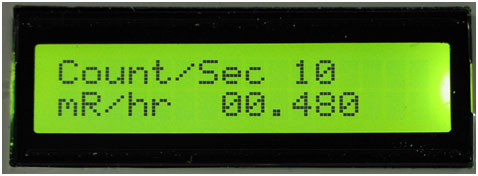Figure 13 shows a digital display from a GCA-07, the Counts Per Second (CPS) on the top line of the LCD. The second line shows the equivalent radiation level. This second line's equivalent radiation level is a running three second average. This three second average smooths out the reading so it's not jumping every second in response to the changes in the CPS.

Imperial or Metric Measurement(s)

The digital display also allows you to change from Imperial measurements (mR/hr) to Metric measurements (mSv/hr) with a flip of a switch. Figure 14 shows a digital display from a GCA-07, the Counts Per Second (CPS) on the top line of the LCD as before. The second line shows the equivalent radiation level using metric measurements of mSv/hr. This second line's equivalent radiation level is also a running three second average, as explained before.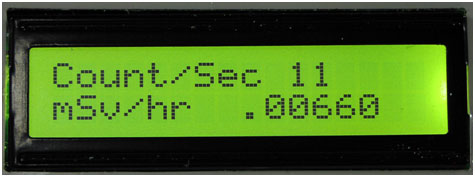Figure 14 Switch from Imperial to Metric Measurements

CPM Measurments:

When switching to the CPM mode the digital screen changes, see figure 15.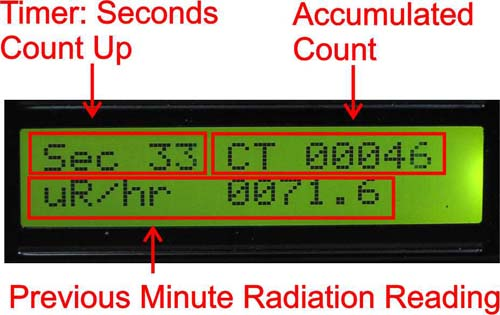Figure 15
The count down tracks how many seconds have elapsed in the current time period measurement. The count is the accumulated count of the number of radioactive particles detected. The second line shows the previous minute radiation level.

One can also switch from Imperial measurement uR/hr to metric measurement uSv/hr in CPM mode.

Figure 16 shows a count that has just started.

Figure 17